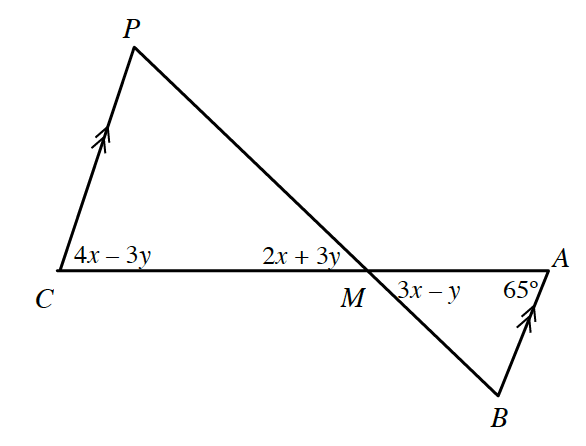### Home > INT3 > Chapter 8 > Lesson 8.1.1 > Problem8-14

8-14.

What is the measure of $∠CPM$ in the diagram at right? List any subproblems that were necessary to solve this problem.

Recall the angle relationship between vertical angles.
Can you isolate one variable on one side of the equation?

If lines $CP$ and $AB$ are parallel, then what is true about angles $C$ and $A$?

Solve the system:
$2x + 3y = 3x − y$
$4x − 3y = 65^\circ$

Once you know the values of $x$ and $y$, determine the angles in the triangle.

$60^\circ$